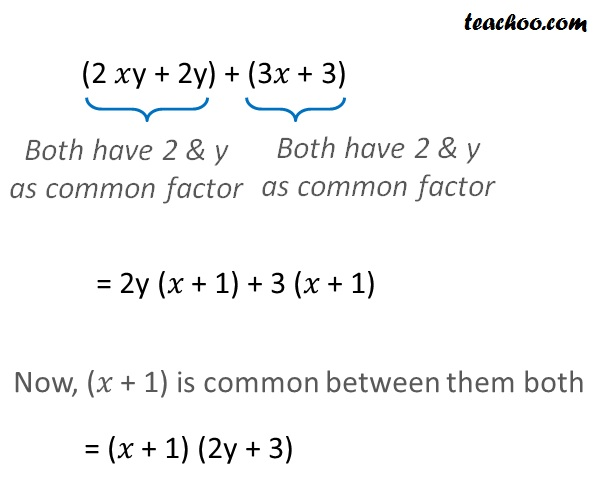Factorisation by regrouping terms

Chapter 12 Class 8 Factorisation
Concept wise

Suppose we want to factorise,

2 x y + 3 x + 2y + 3

We note that,

2xy & 2y both have 2

And

3x & 3 both have 3

So, we put them together

(2xy + 2y) + (3𝑥 + 3)So,

2𝑥y + 3𝑥 + 2y + 3 = (𝑥 + 1) (2y + 3)

### Factorise 4ab − 5a − 12b + 15Learn in your speed, with individual attention - Teachoo Maths 1-on-1 Class

### Transcript

(2𝑥y + 2y) + (3𝑥 + 3) Both have 2 & y as common factor Both have 2 & y as common factor = 2y (𝑥 + 1) + 3 (𝑥 + 1) Now, (𝑥 + 1) is common between them both = (𝑥 + 1) (2y + 3) So, 2𝑥y + 3𝑥 + 2y + 3 = (𝑥 + 1) (2y + 3) Factorise 4ab − 5a + 12b + 15 4ab − 5a + 12b + 15 = (4ab − 12b) + (15 − 5a) Both have 4 & b as common factor Both have 3 as common factor = 4b (a − 3) + 5 (3 − a) Writing (3 − a) = −(a − 3) = 4b (a − 3) − 5 (a − 3) Taking (a − 3) common = (a − 3) (4b − 5)